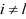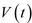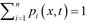In this case                      , where the components of              are built from rate constants           for the transition from state   to state   . The diagonal elements of matrix            are defined as                                   where            if           and 0 otherwise; and off diagonal terms as                           where              if                and 0 otherwise. The rate constants               have finite positive values (>0) only if transition between states      and      are possible otherwise they are equal to 0. Each component of the vector           is the probability of finding the myosin head in one of its     states at time    and at strain    (Huxley, 1957). The operator           is the material derivative                           , where           is the shortening velocity of the actin filament relative to myosin filament. Note that for simplicity, here we assume uniform shortening velocity along the filaments, i.e.              . This is achieved, without loss of generality, by including a series elastic component equivalent to the elasticity of the filaments and apparently rigid filaments in the overlap region where myosin interacts with actin. The normalized shortening velocity,         , denotes          in half sarcomere lengths per second.

The state transition matrix           , formed exclusively from rate constants           , is singular because the sum of the elements in each column is equal to zero, i.e. determinant                      . Thus, to find a unique solution of Eq. 5, it is necessary to replace one row in              by a constraint equation. In the original Huxley 57 model (Huxley, 1957), the constraint is that at any instant of time    , the sum of all state probability densities at particular     is equal to  1, i.e.                         . This constraint was later used in almost all sliding filament models (Hill, 1974; Pate and Cooke, 1989; Smith and Geeves,1995a, Smith and Geeves,1995b; Mijailovich et al., 1996). Examples of state transition matrices           are shown here.